# OPERATORS FOR OLAP APPLICATIONS

Recently, in the literature, many authors have proposed operators and query languages which refer to OLAP applications. This acronym, coined and proposed in Codd, Codd, & Salley (1993) to characterize the requests for summarizing, consolidating, viewing, applying formulae to, and synthesizing data according to multiple dimensions, resumes the concept of enabling analysts, managers, and executives to gain insight into the performance of an enterprise through fast access to a wide variety of views of data organized to reflect the multidimensional nature of the enterprise data (Colliat, 1995). The previous approaches were the basis for the new operator proposals in the OLAP environment, changing the goals of the users, which focused greater attention on the dynamic analytical processing of data on-line, and therefore on the consequent problems linked to this application, such as access performance, or the number of materialized views to store. Depending on the initial approach, relational or multidimensional-tabular, the relative processes have been called ROLAP (Relational On-Line Analytical Process); see MicroStrategy (http://www.strategy.com), MOLAP (Multidimensional On-Line Analytical Process), and ArborSoft (arborsoft.com). As discussed in Shoshani (1997), MOLAP proponents claim that:

1. Relational tables are unnatural for multidimensional data.

2. Multidimensional arrays provide efficiency in storage and operations.

3. There is a mismatch between multidimensional operations and SQL.

4. For ROLAP to achieve efficiency, it has to perform outside current relational systems, which is the same as what MOLAP does.

1. ROLAP integrates naturally with existing technology and standards.

2. MOLAP does not support ad hoc queries effectively, because it is optimized for multidimensional operations.

3. Since data has to be downloaded into MOLAP systems, updating is difficult.

4. Efficiency of ROLAP can be achieved by using techniques such as encoding and compression.

The claim that MOLAP performs better than ROLAP is intuitively believable, and in Zhao, Deshpande, & Naughton (1997), this was also substantiated by tests.

Now we examine the most relevant proposals, presenting, if necessary, the reference model (the data models have been discussed in Chapter 2).

In Gray et al. (1996), and subsequently in Gray et al. (1997), the authors propose the data cube operator as an extension to SQL which generalized the histogram, group-by (the classic SQL operator extended to support histograms and other function-valued aggregations), cross-tabulation (the 2-D data cube obtained crossing two category attributes of the n-dimensional cube structure), roll-up (going up the levels of a hierarchy), and drill-down (going down the levels of a hierarchy) constructs found in most reports. An example of these constructs is shown in Figure 2 (the original figure appears in Gray et al., 1997).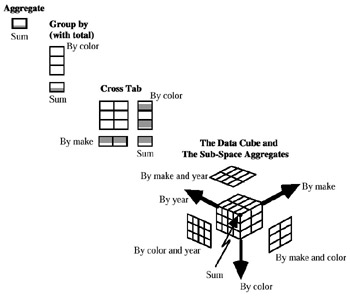Figure 2

In Li & Wang (1996), the authors formalized a multidimensional data model for OLAP, and developed an algebra query language called Grouping Algebra which is the basis for a multidimensional cube algebra, proposed in order to facilitate the data derivation.

After the definition of the classic rename, roll, and aggregation operators, the authors introduce other operations, namely: add dimension (which generates a new cube which has a new dimension named D, whose relation scheme is the empty set, and the relation for the dimension only has one tuple, i.e., the empty tuple), transfer (which generates a new cube by transferring attribute A of dimension D1 to a (new) attribute B on dimension D2), union, cube aggregation (which generates a new cube by "compressing" the original cube into a smaller one), rc-join (which joins the relation r to dimension D1), and construct (which generates a cube from relation r so that the new cube is one-dimensional).

In Gyssens & Lakshmanan (1997), the authors give a foundation for multidimensional databases. They define a slicing and dicing operation as "just a special case of relational selection extended to multidimensional data tables," and also propose the classic algebraic operators. In particular, they define three sets of classic algebraic operators. The first consists of three unary operators, selection σC, projection πX, and renaming ρB A, where C is a valid selection condition, defined as usual, X is a subset of the attribute set of the table (i.e., the MAD), and A and B are attribute names. The authors define op(τ) = tabS(op(rep(τ)), where they denote a table instance by τ, its tabular representation by tabS (r) (i.e., a table instance with schema S), and the relational representation f(τ) of the table by rep(τ). After having discussed the classic operators union, intersection, difference, and Cartesian product, they propose the following operators: fold (which reduces the table by one dimension, modifying the table schema), unfold (the inverse of the previous one), classification (defined on relations and on tables, it maps tuples of a relation to one or more groups), and summarization (where the structure of the output table is the same as the input table, as far as the dimensions and parameters are concerned; the only change is that sets of measure tuples are summarized according to the aggregate function and transformed into single values). Finally, they give a rigorous formal expression of data cube and monotone roll-up.

In Agrawal, Gupta, & Sarawagi (1997), the authors propose a data model which provides support for multiple hierarchies along each dimension and for ad hoc aggregates, as well as a few algebraic operators. In this paper, they deal more with multiple hierarchies, and introduce the multiplicity of a hierarchy as a semantic variant of the simple one. The operators which they propose are: push (it converts dimensions into elements that can be manipulated using function felem), pull (it is the converse of the push operator, and creates a new dimension for a specified member of the elements), destroy dimension (this operator removes a dimension D that had a single value in its domain), restriction (this operator operates on a dimension of a cube and removes the cube values of the dimension that do not satisfy the initial condition), join (it is used to relate information in two cubes; the result of joining an m-dimensional cube with an n-dimensional cube on k dimensions is cube C with n+mk dimensions), the classic Cartesian product, natural join, union, merge (this is an aggregation operation which maps multiple product names to one or more categories, or similar mapping), and associate (depending on the associative function felem, it transforms the measure values of two cubes into another cube, with possible reductions of the domain instances of the dimensions, and the possible changing of the summary type of the cube).

In Cabibbo & Torlone (1997, 1998), the authors propose a logical model for OLAP systems that is usable in designing multidimensional databases, and independent of any specific implementation. The operators proposed in this paper are the classic Cartesian product, natural join, renaming, selection, (simple) projection, roll-up, aggregation, as well as level description, Scalar function application, and abstraction.

In Lehner (1998) the author discusses the design problem which arose when the OLAP scenarios became very large, and proposes a nested multidimensional data model useful during schema designing and multidimensional data analysis phases. The author points out that he will not propose another data model, but provide necessary extensions in different directions. After having defined the nested multidimensional data cube, he introduces operators on multi-dimensional objects (MOs). In particular, they have the slicing operator (it produces a new MO which inherits all components of the source MO except the context descriptor), drill-down, roll-up, split, merge, explicit aggregation, and cell-oriented operators (which are the classic unary operators , abs, and sign, and binary operators *, /, +, , min, max, and which work directly on cells).

In Nguyen, Tjoa, & Wagner (2000), the authors propose a conceptual multidimensional data model based on MetaCube, and three basic navigational metacube operators which are applied to navigate along a metacube C, corresponding to a dimension D, namely jumping, rollingUp and drillingDown.

In Pedersen & Jensen (1999), and subsequently in Pedersen, Jensen, & Dyreson (2001), after a survey of 14 multidimensional data models, the authors propose an extended multidimensional data model and algebraic query language. Then, they define the basic algebra of the model, where the operators are similar to the standard relational algebra operators. In particular, they propose group (which groups the facts characterized by the same dimension values), union (which performs a union of the categories and the partial orders, taking the set union of the facts and the fact-dimension relations), selection (which restricts the set of facts to those that are characterized by values where a given predicate p on the dimension types evaluates to true; dimensions and schema stay the same), projection (over k dimensions, without removing duplicate values), rename (used to alter the names of dimensions), difference (which obtains the set-difference of the facts, while the dimensions of the first argument are retained, and the fact-dimension relations are restricted to the new fact set), identity-based join (the new fact type is the type of pairs of the old fact types, while the new set of dimension types is the union of the old sets; the set of facts is the subset of the cross-product of the old fact sets, where the join predicate p holds), aggregate formation (see Klug, 1982), value-based join (a join between two MADs on common dimension values, by combining Cartesian product, selection, and projection), duplicate removal (elimination of "duplicate values," i.e., facts characterized by the same combination of dimension values), SQL-like aggregation (it carries out the computation of an SQL aggregate function on a MAD grouped by a set of dimension categories, by first applying the aggregate formation operator with the given categories and the given function), star-join, drill-down (it gives more detail by descending the dimension hierarchies), and roll-up (it gives less detail by ascending, the dimension hierarchies). They also propose two operators for handling time, namely valid-timeslice and transaction-timeslice.

As noted in Shoshani (1997), there is a terminology correspondence between the multidimensional aggregate (statistical) databases and OLAP areas. The more common equivalent terms are:

 Category Attribute Dimension Category Hierarchy Dimension Hierarchy (table) Category Value Dimension Value Summary Attribute Measures (fact column) Statistical Object Data Cube (fact table) (Multidimensional Aggregate Data) Cross-Product Multidimensionality Summary Table Table/Data Cube

Before speaking of the operators—taken mainly from the set of all the operators previously presented, which will form the base algebra, together with other operators which, even if not strictly necessary, enlarge the base algebra in order to facilitate the definition of multidimensional aggregate query languages—we return to the symmetric treatment of dimension and measure of a MAD. This is for two reasons: i) in order to demonstrate that this symmetric treatment was also possible (even if not always necessary) in a statistical database, and ii) that the problems which arose in a statistical database repropose word for word in an OLAP environment. It is evident that a "described" data can become, in its turn, a "describing" data, i.e., to play either the role of parameter (or category attribute) or the role of measure (or summary attribute). Therefore, a measured data is not always also a "summary" data. Problems arise when, after an exchange between the measure (summary) and one dimension, we apply the same operators which normally apply to the original conditions.

For example, suppose we have the table shown in Figure 3.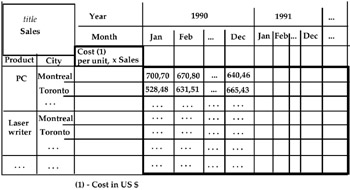Figure 3

In reality this table does not have aggregate data, i.e., it is a classic relation, as can be seen in Figure 4.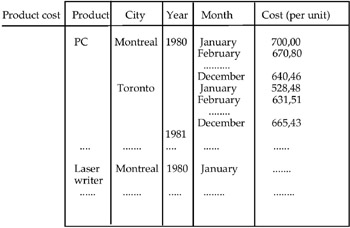Figure 4

As can be easily seen, the only change is the different way of representing the data. In this situation an exchange between "Cost (per unit)" and another attribute (for example, "Product") is also easy to carry out. The more evident problem is the large quantity of null values which appear as "measure," as can be seen in Figure 5.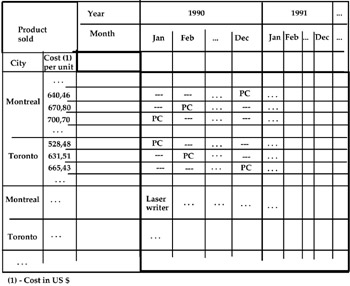Figure 5

Different problems arise when we try to apply the aggregate operators to this relation. For example, if we group the instances of the attribute "Month" in the table in Figure 3 by the value-range "Quarter," we do not know what the PC cost is in the different cities in the first quarter, or the second quarter, etc. At the same time, in the table in Figure 5, if we group the "Cost per unit" values into two value-ranges, "650" and ">650" respectively, and then we apply the roll-up operator to the hierarchy Year-Month, the PC instance of the attribute "Product" appears in both the rows "650" and ">650" relative to city = Montreal, as shown in Figure 6.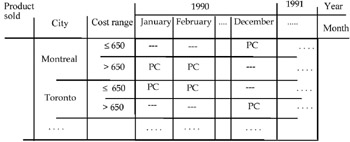Figure 6a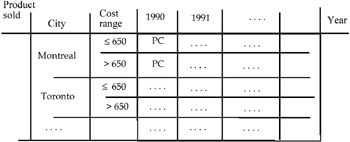Figure 6b

Analogous problems happen if we only use the operator roll-up applied, for instance, to the hierarchy "Year-Month." In fact, if we delete the attribute "Month" in Figure 3, what is the cost measured in 1990, in 1991, and so on ? If instead we have a MAD, i.e., an array with a measure obtained by an aggregation process and, therefore, as a first result, numbers as measures in the cells of the MAD, we can still exchange the numeric measure with another dimension.

Applying the group-by operator on this new MAD, some inconsistencies can be produced. For example, if we group into two ranges ("15" and ">15"), the values relative to "Sold Qty," the measure "Vendor" appears in the cells as shown in Figure 9(a).

If, then, we group the cities again by region, we can have two different situations: i) the vendors B and C appear both in the row "15" and in the row ">15."

In this case it is very difficult to define a general rule by which if B (or C) is defined by two (or more) different values of the same dimension, it is automatically defined only by the greater value (in this case, ">15"). Also, the vendor A is described only by one value of a dimension (in our case, "15").

In this case it is impossible to find a general rule by which if A is defined by only one value of a dimension, then applying the grouping operator to another dimension, it remains as described by the same previous value.

For example, if A has sold 15 IBM computers in all the cities of the region Lazio, grouping these cities in region = Lazio, it is impossible to understand if the total number of IBM computers sold by A in Lazio is still 15 or not (see Figure 9(b)). This is analogous for the vendor C and Macintosh computers.

Suppose we consider the MAD in Figure 7. Suppose we also exchange "Vendor" with "Sold quantity." The new MAD is shown in Figure 8.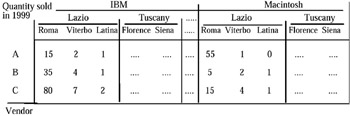Figure 7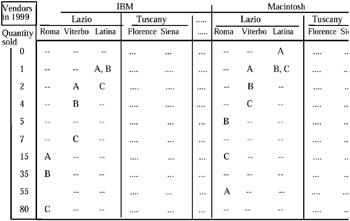Figure 8

This means that it is always possible to exchange a numeric measure (obtained by an aggregation process from microdata) with a dimension, but on the new MAD it is very often impossible to operate again with the classic operators for aggregate data without risking having inconsistent or wrong data.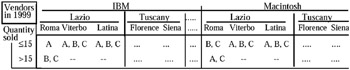Figure 9a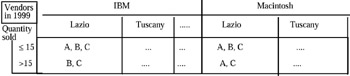Figure 9b

This observation is implicitly confirmed in Hurtado, Mendelzon, & Vaisman (1999), where the authors note that in a relational implementation of OLAP (namely ROLAP), facts (viewed as a mapping from a point in a space of dimensions into one or more spaces of measures) are stored in fact tables, while each dimension is described in a dimension table. The usual assumption is that fact tables (generally summary data) reflect the dynamic aspect of the database (or, in general, of a data warehouse), while data in dimension tables (the descriptive space) are relatively static.Multidimensional Databases: Problems and Solutions
ISBN: 1591400538
EAN: 2147483647
Year: 2003
Pages: 150